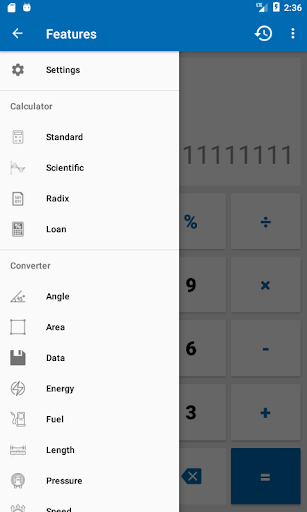NT Calculator – Extensive Calculator Pro v2.8 [Paid] – Android Applications

NT Calculator – Extensive Calculator Pro v2.8 [Paid]
Requirements: 4.4+
Overview: This Extensive Calculator app allows you to easily handle all the calculations necessary for everyday life with a single application. A Calculator app with a simple interface and practical functions! It can replace your default calculator.With Extensive Calculator Pro on your Android, you do not need to bring your calculator anymore. This calculator app serves you everything from A – Z.
List of calculators currently supported:
1. Standard Calculator
• Quick and easy.
• History available.
• Percentage Calculator: 100 – 30% – 70
• Simple interface
• Basic features such as: %, +, -, x, /, 0 – 9, ( and ).
Ex: -(2+4)x4 = -24
2. Scientific Calculator
• Basic arithmetic operations including percentage, modulo and negation;
• History available.
• Supports the four fundamental arithmetic operations, square, formula’s parenthesis, and simple scientific operations such as trigonometric and logarithmic functions.
• All features such as: sin, cos, tan, %, !, 0-9, (, ), RAD and DEG, log, ln, Mod, +, -, x, /, SQRT, SQR, signed,…
• In scientific calculator, It has a button to change RAD to DEG and contrary.
• You can use EXP is E (sam as 10 ^ ) to calculate large decimal number. If you need to get previous result of calculator, you can use ANS button.
3. Loan Calculator
• Simple interface
• Suitable for car, bank loan
• Three primary input are loan amount, interest rate, loan term.
• Loan calculator show you the amount paid per month and total interest.
4. Converter
Supports many Unit of measurement commonly used in everyday life such as:
• Weight converter: kg to st, lbs to grams, lbs to kilograms, stones to grams,…
• Length converter: size converter, meters to kilometers, mile in km, …
• Speed converter: km/h, cm/s, m/s, m/s to km/h, …
• Temperature converter: c to f, f to c, c to kelvin, …
• Data converter: byte to bit, bit to byte, …
• Area converter: km2 to m2, 1 hectare to km2, cm2 to m2, mm2 to m2, …
• Time converter: minute to second, year to second, millisecond to second, …
• Energy converter: joule, kwh, …
• Pressure converter: atmospheres, bars, kilopascals, pascals, millimeters of mercury, pound per square inch
• Volume converter: gallons to qts, teaspoon, cups, liters, cubic feet, quarts, pints, fluid ounces (US and UK),…
• Fuel Converter: many units,…
Thank you for using the Calculator app, if you have questions I am always ready to answer you. I think you will find this app useful and use it with pleasure.
The list will be increased!

What’s New

– New function: Time calculator.
– You can use the calculation again by clicking on any line in the history.
– Do you want to copy? Hold the place to copy.

`https://play.google.com/store/apps/details?id=thanhletranngoc.calculator.pro`